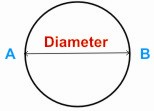HOME MATH DICTIONARY DOWNLOAD FEEDBACK DISCLAIMER
 Question: What is a Diameter ? Answer: A diameter of a circle is a straight line through its center which joins two points on its circumference, such as A and B. Its length is twice that of the radius. Each diameter is a line of symmetry for the circle and cuts it into two semi-circles. The diameter is the longest chords of the circle. In more modern usage, the length of a diameter is also called the diameter. In this sense one speaks of the diameter rather than a diameter, because all diameters of a circle have the same length, this being twice the radius. Example of a Diameter: